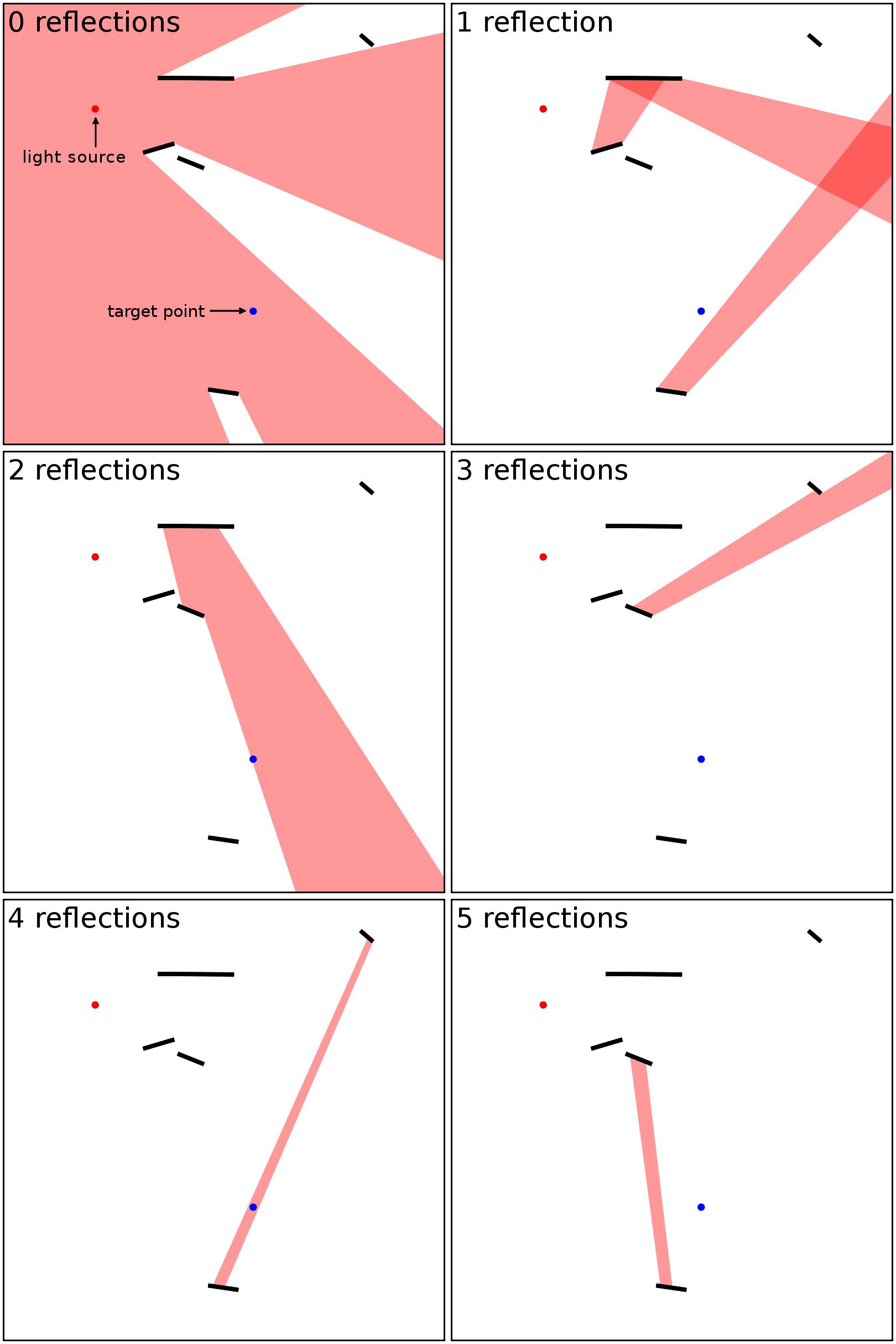CSES - HIIT Open 2016 - Light and mirrors
• Time limit: 2.00 s
• Memory limit: 256 MB
There are a light source, a target point and five mirrors on a two-dimensional plane. Your task is to find out whether a ray that originates from the light source can reach the target point through exactly $0,1,\ldots,5$ reflections.

The light source sends rays to all directions. All mirrors are double-sided, i.e., a ray will reflect from both sides of the mirror.

Input

The first input line contains an integer $t$: the number of test cases. After this, the test cases are described as follows:

First there are two lines that specify the locations of the light source and the target point. Both lines contain two integers $x$ and $y$, meaning that the location of the point is $(x,y)$.

Finally, there are five lines that specify the locations of the mirrors. Each line contains four integers $x_1$, $y_1$, $x_2$ and $y_2$, meaning that a there is a mirror between points $(x_1,y_1)$ and $(x_2,y_2)$.

Output

For each test case, output a binary string $x_0x_1\ldots x_5$ where $x_i$ is $1$ if light reaches the target through $i$ reflections.

Constraints
• $1 \le t \le 10$
• all input coordinates are between $0 \ldots 10^9$
• the result does not change if the input coordinates are changed by at most one
• no three input points are collinear
• no mirrors intersect
Example

Input:
1 209000000 760000000 566000000 303000000 317000000 661000000 388000000 682000000 533000000 116000000 464000000 126000000 523000000 829000000 350000000 830000000 808000000 928000000 837000000 903000000 455000000 626000000 395000000 650000000

Output:
101010

The following figures show the setting in the example, and the areas that are reachable through $0,1,\ldots,5$ reflections: